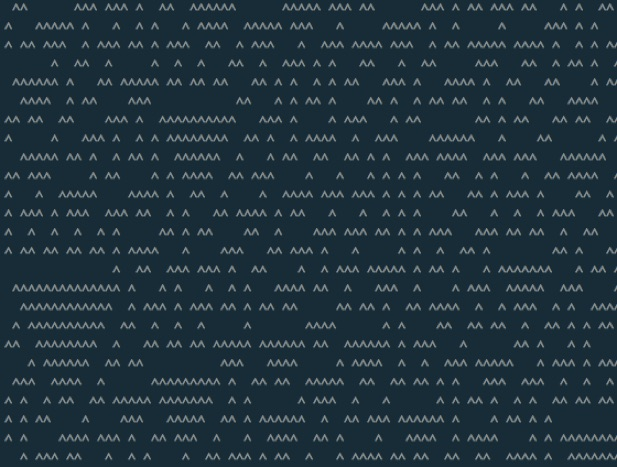Rule 30 was created in response to a call for works. The requirements specified that the code must be smaller than 512 bytes and must compile. My response was a generative, concrete poem. The title and visual aesthetic both refer directly to Stephen Wolfram’s cellular automata series. At left is the code poem. Below is one frame of the animated concrete output. The computational sublime is found in the never-ending visual generation. Note that while appearing random, this one-dimensional cellular automata is not random and will reproduce exactly the same concrete poem every time. Rule 30 is included in code {poems}.

You may or may not additionally need the -ansi flag to compile.

```//c++ rule30.cpp -o rule30
#include
int i,j,l,r,o={},t={};
char c;
int main(){
std::cout<<"enter character, then return >> ";
std::cin>>c;
o=1;
while(1){
for(j=0;j<80;j++){
if(j)     l=o[j-1]; else l=o;
if(j!=79) r=o[j+1]; else r=o;
if(o[j])
if(l)   t[j]=0;   else t[j]=1;
else
if(l^r) t[j]=1;   else t[j]=0;
std::cout<<((o[j]?" ":&c));
}
for(;--j>=0;) o[j]=t[j];
std::cout.flush();
usleep(50000);
}
}```